Chapter 3 Part 2 - Banking

Economics Class 12
Macroeconomics

##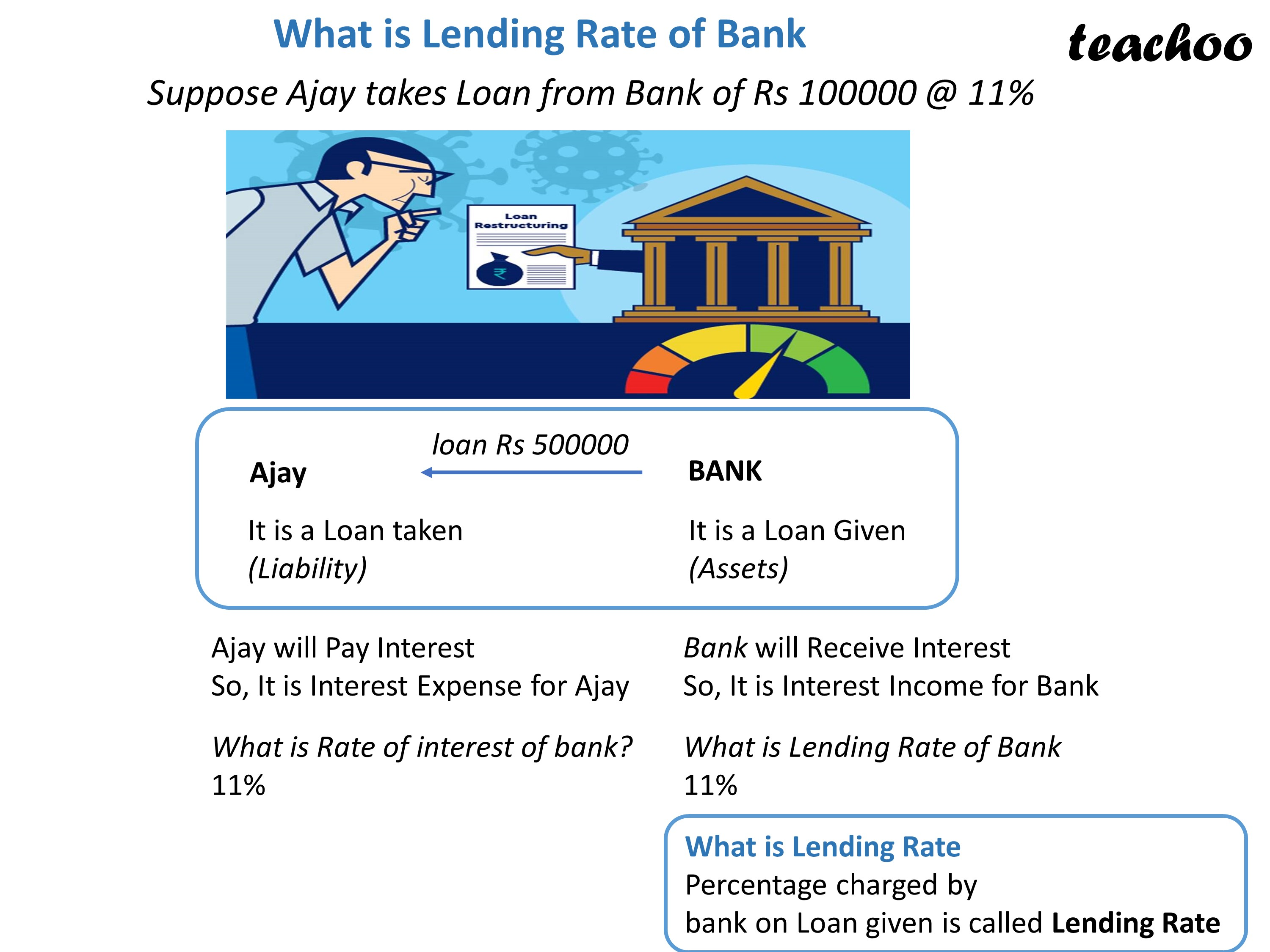googletag.cmd.push(function() { googletag.display('div-gpt-ad-1669298377854-0'); });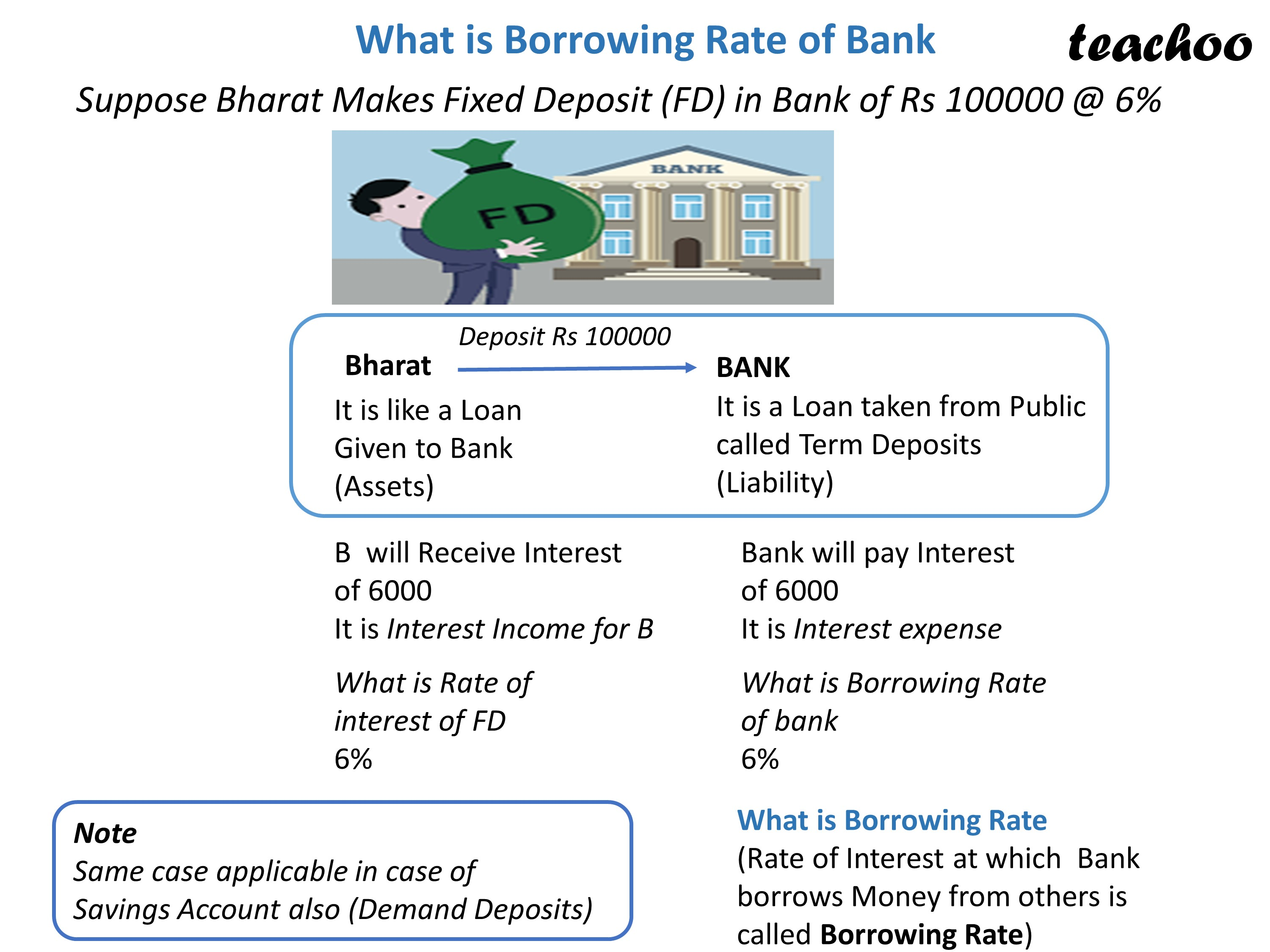## How does a Bank earns money?

A bank takes money from public at low rate of interest

It gives loan to Public and businessman at high rate of interest

Remaining is its income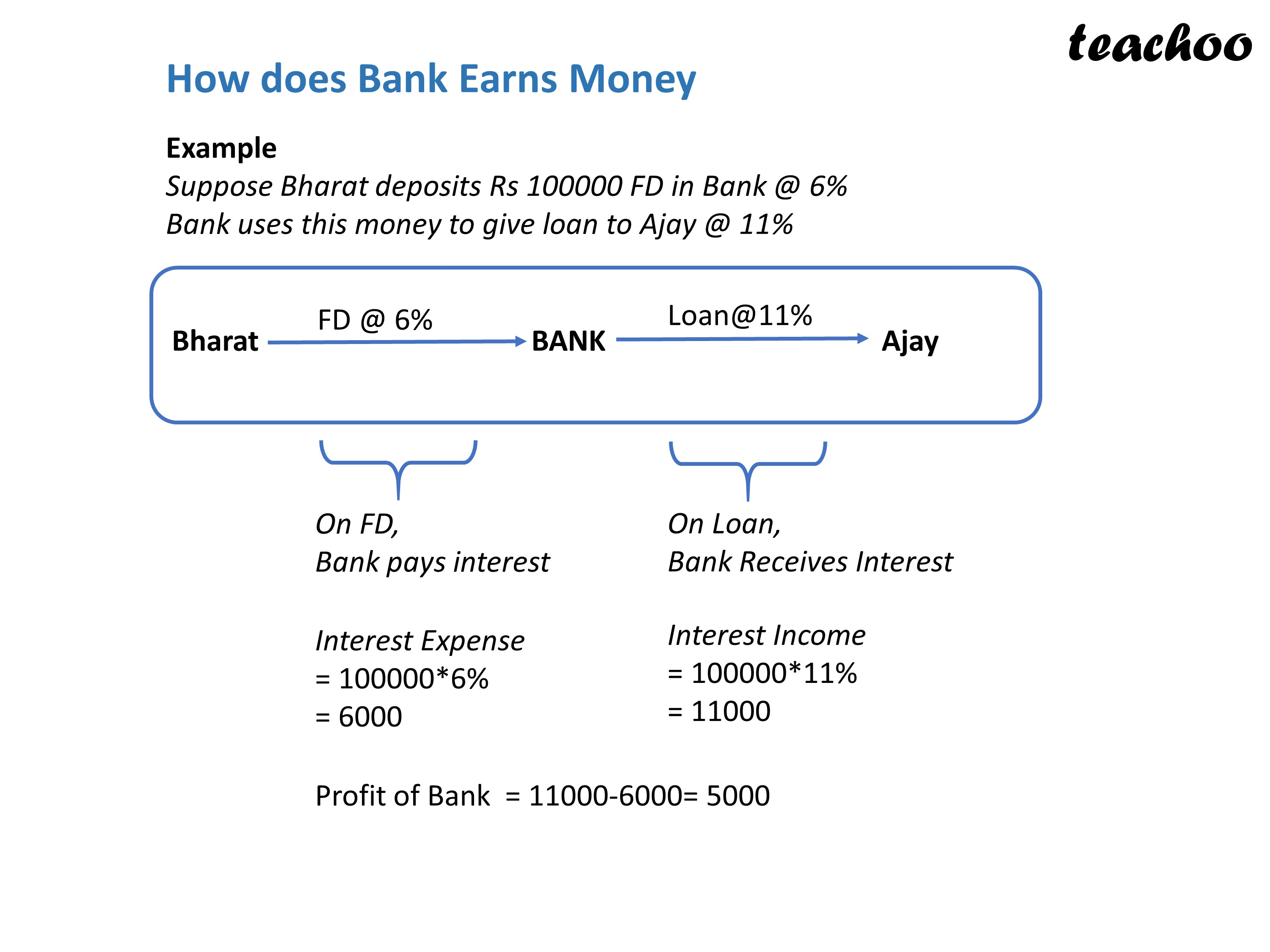## What is borrowing Rate of Bank in this case?

Borrowing Rate of banks is Rate of Interest offered by banks to deposit holder

Borrowing Rate in our example = 6%

## What is Lending Rate of Bank in this case?

Lending Rate is the Rate charged by bank on lending money

Lending Rate in our example = 11%

Spread means Difference between Lending Rate and borrowing Rate

Spread in our case = 11% - 6% = 5%

Note

More the spread, more the profit of bank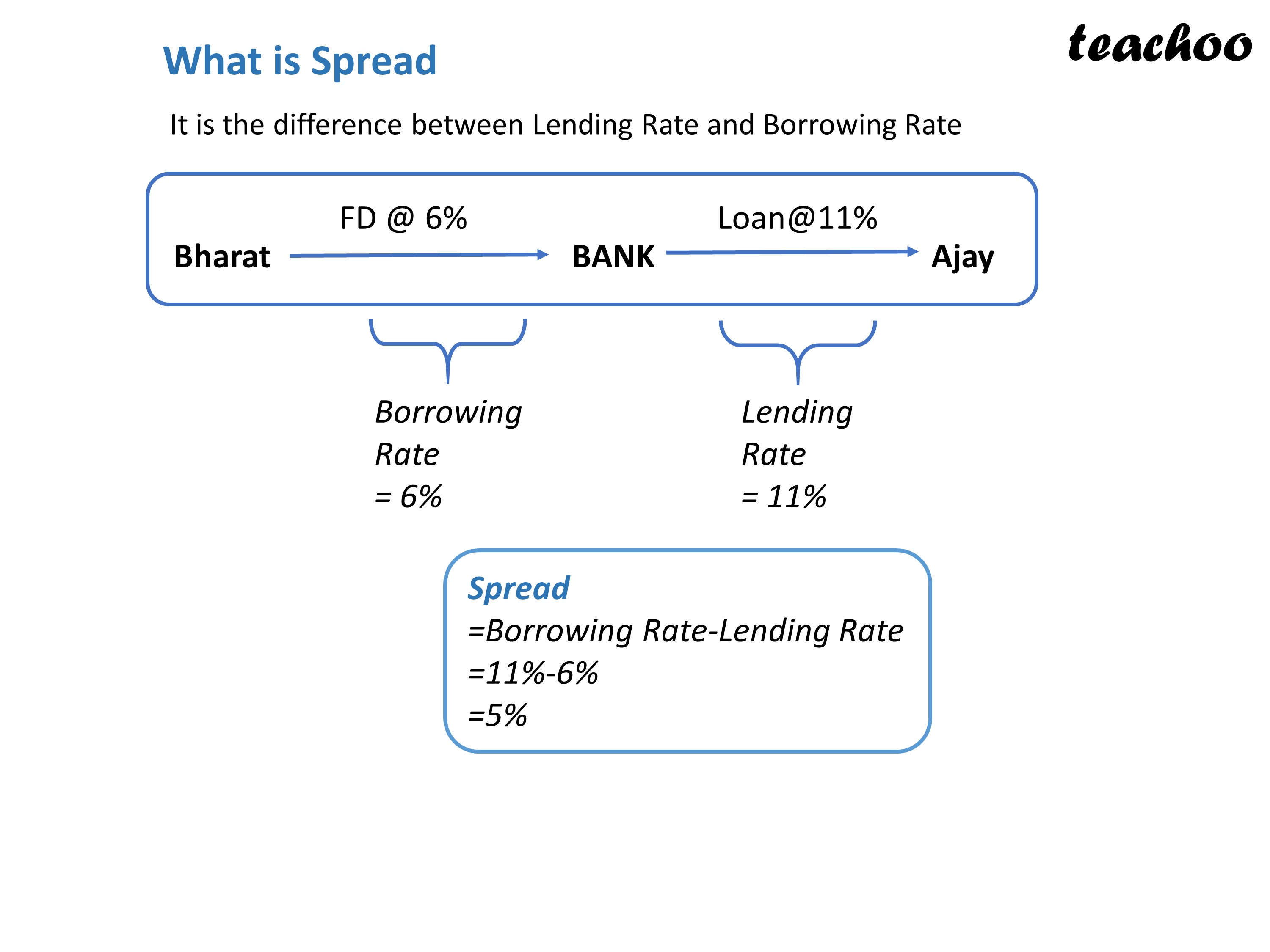## What does bank check while giving loans?

Bank checks

Credit worthiness of person (whether person will be able to repay loan)

Collateral (Security in form of property offered)

Repayment Capacity

Financial Ratios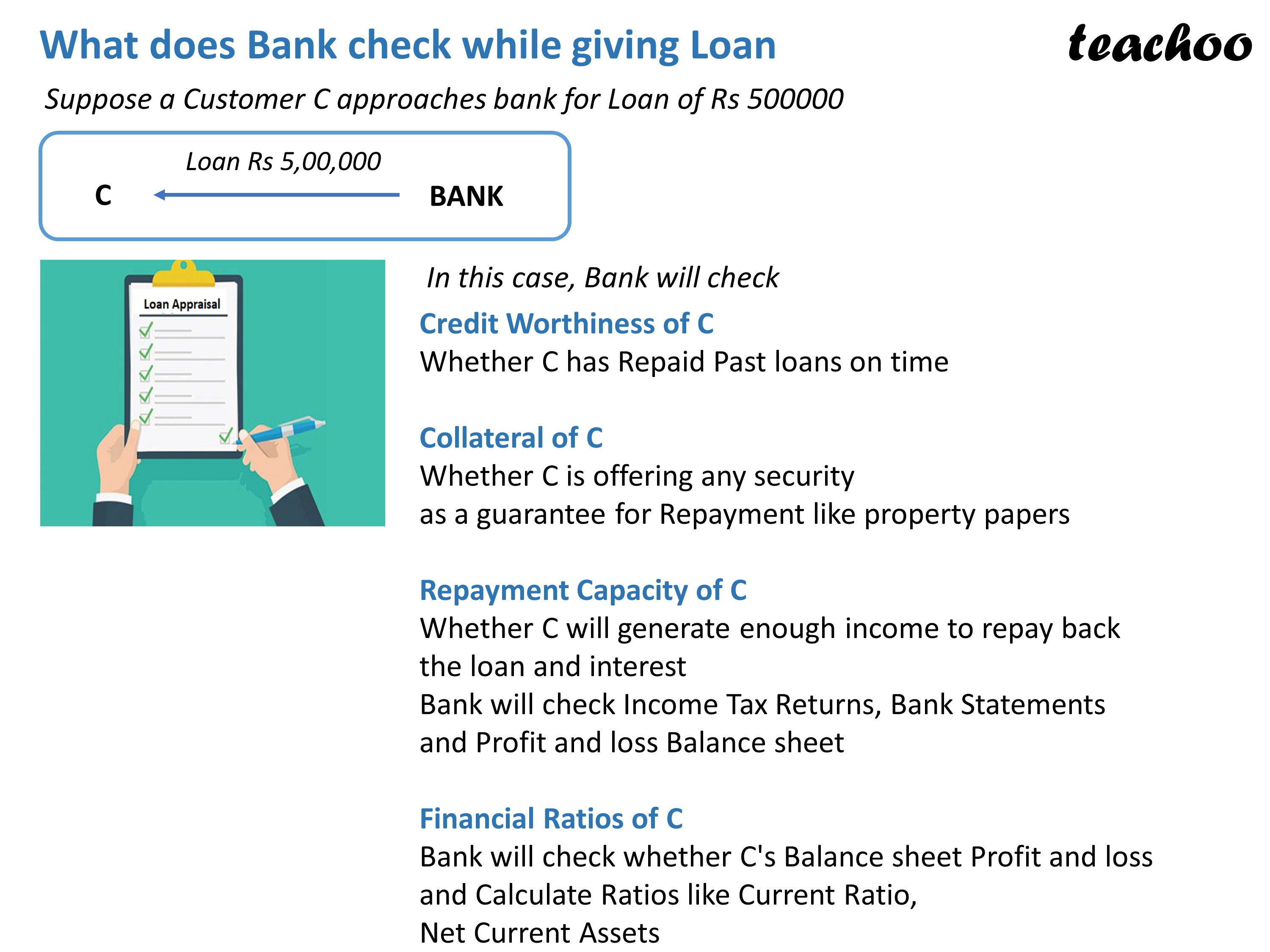Learn in your speed, with individual attention - Teachoo Maths 1-on-1 Class

### Transcript

What is Lending Rate of Bank Suppose Ajay takes Loan from Bank of Rs 100000 @ 11% loan Rs 500000 It is a Loan taken (Liability)It is a Loan Given (Assets) Ajay will Pay Interest So, It is Interest Expense for Ajay What is Rate of interest of bank? 11% Bank will Receive Interest So, It is Interest Income for Bank What is Lending Rate of Bank 11% What is Lending Rate Percentage charged by bank on Loan given is called Lending Rate What is Borrowing Rate of Bank Suppose Bharat Makes Fixed Deposit (FD) in Bank of Rs 100000 @ 6% It is like a Loan Given to Bank (Assets) is a Loan taken from Public called Term Deposits (Liability) B will Receive Interest of 6000 It is Interest IncomeBank will pay Interest of 6000 It is Interest expense What is Rate of interest of FD 6%What is Borrowing Rate of bank 6%What is Borrowing Rate (Rate of Interest at which Bank borrows Money from others is called Borrowing Rate) Note Same case applicable in case of Savings Account also (Demand Deposits) How does Bank Earns Money Example Suppose Bharat deposits Rs 100000 FD in Bank @ 6% Bank uses this money to give loan to Ajay @ 11% On FD, Bank pays interest On Loan, Bank Receives Interest Interest Expense = 100000*6% = 6000Interest Income = 100000*11% = 11000 Profit of Bank = 11000-6000= 5000 What is Spread It is the difference between Lending Rate and Borrowing Rate Borrowing Rate = 6% Lending Rate = 11% Spread =Borrowing Rate-Lending Rate =11%-6% =5% What does Bank check while giving Loan Suppose a Customer C approaches bank for Loan of Rs 500000 In this case, Bank will check Credit Worthiness of C Whether C has Repaid Past loans on time Collateral of C Whether C is offering any security as a guarantee for Repayment like property papers Repayment Capacity of C Whether C will generate enough income to repay back the loan and interest Bank will check Income Tax Returns, Bank Statements and Profit and loss Balance sheet Financial Ratios of C Bank will check whether C's Balance sheet Profit and loss and Calculate Ratios like Current Ratio, Net Current Assets